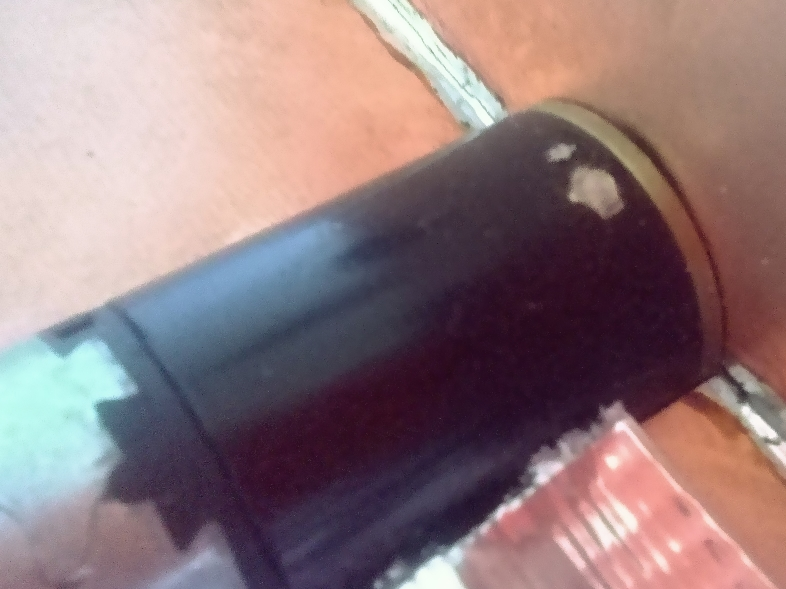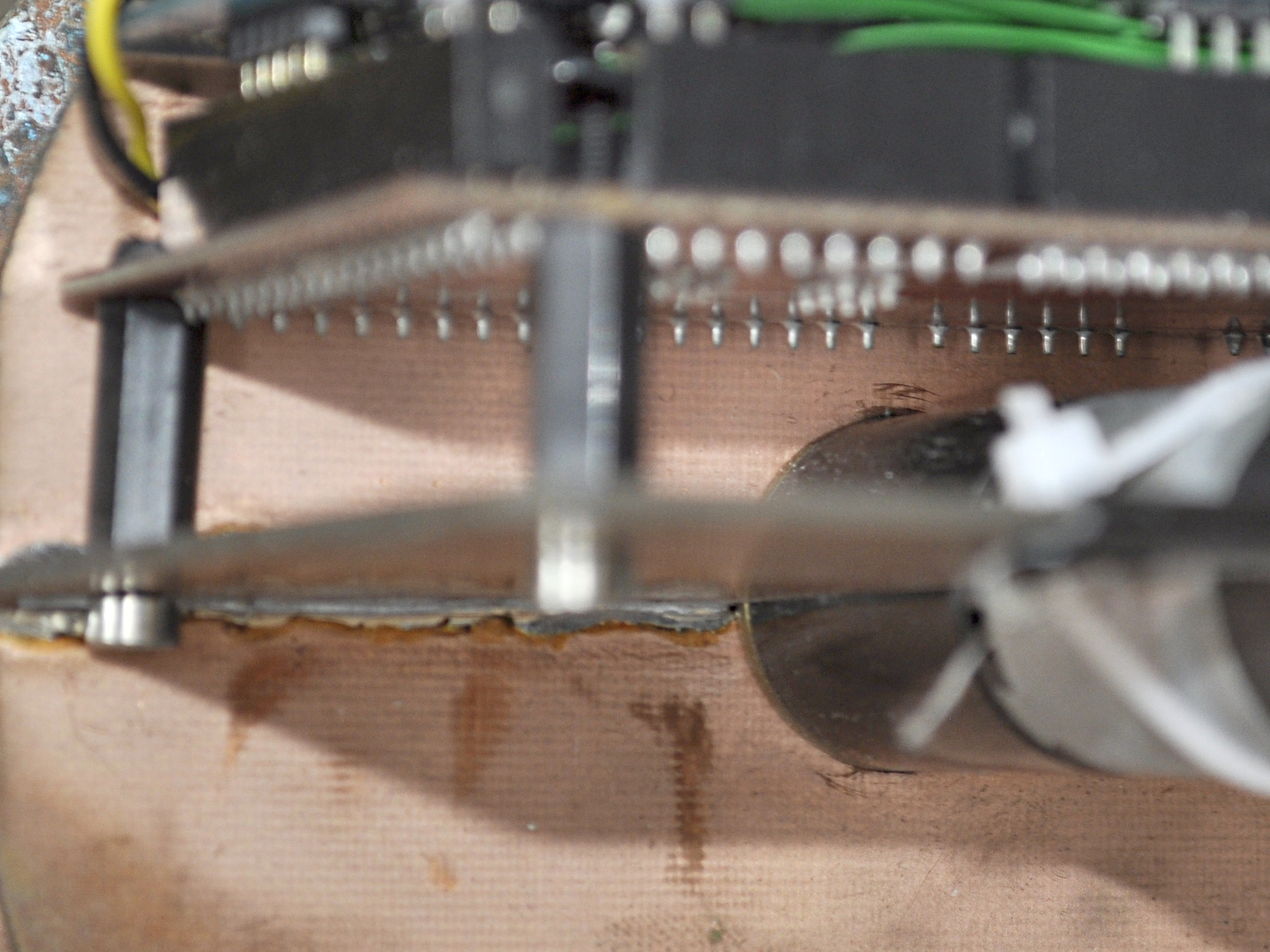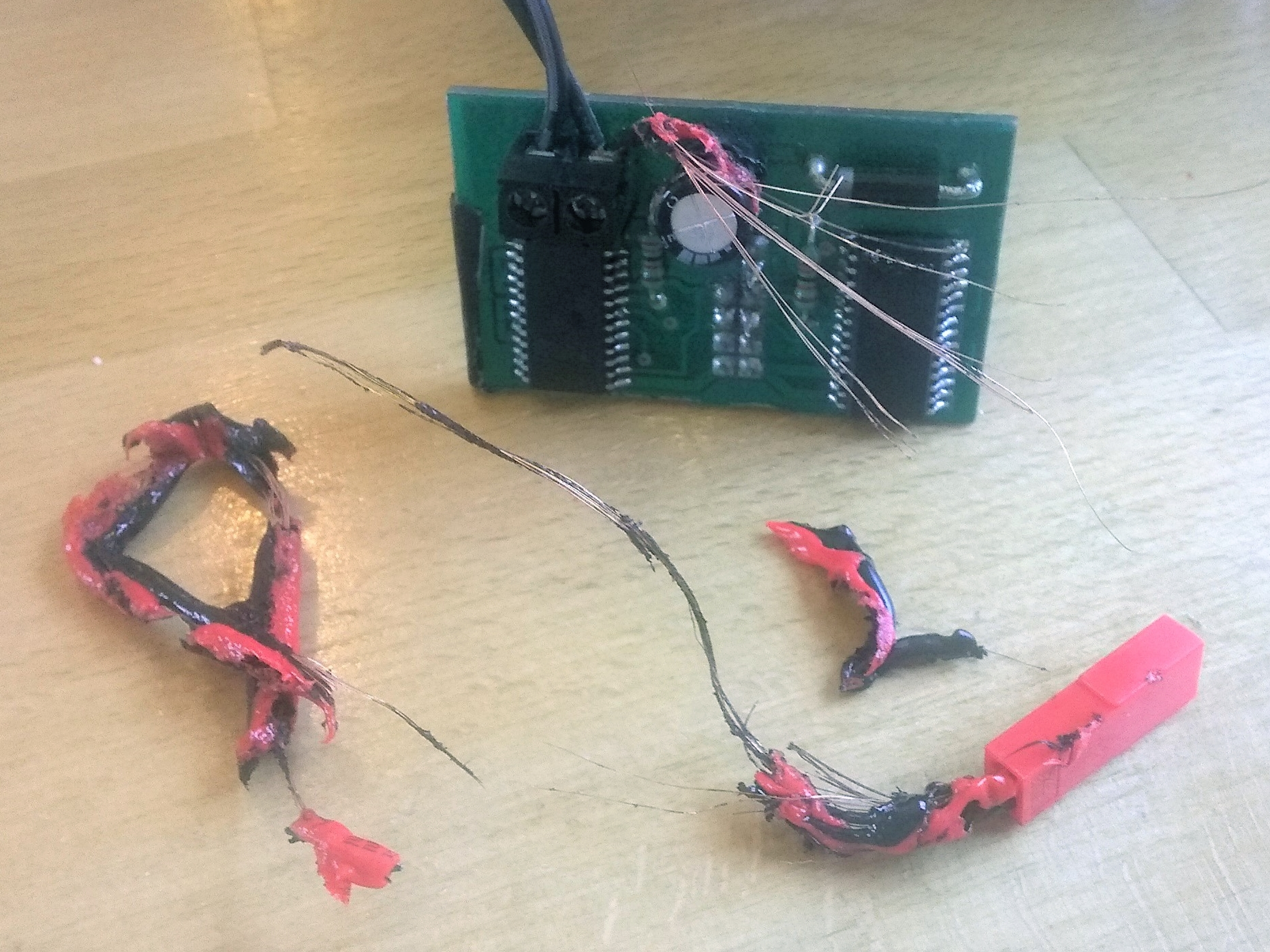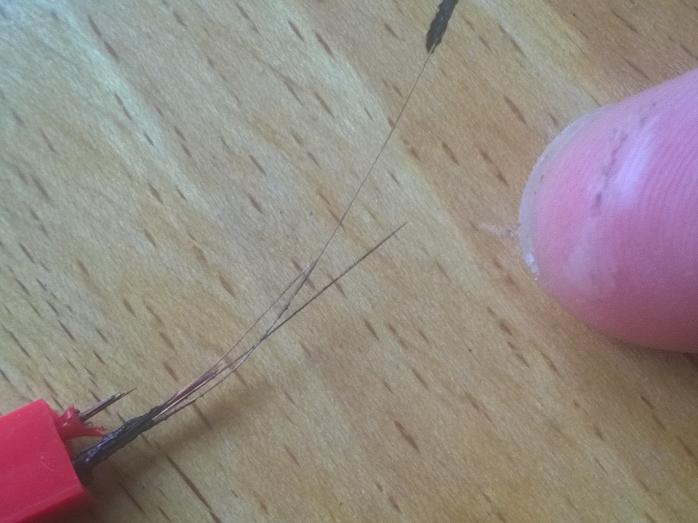$\newcommand{\tind}{^{(#1)}} \DeclareMathOperator*{\argmin}{arg\,min} \newcommand{\bm}{\mathbf{#1}}$

# Machine learning for a miniature robotic unicycle

Eric Wieser (efw27@)

MEng. Project 2016-2017
Supervised by Prof. Carl Rasmussen (cer54@)

## Project goals

Balance this unicycle

Fix problems identified in previous work

Review source code and fix bugs found

Improve the tools to make similar projects easier in future

## Outline

1. System overview
2. Summary of the method, Pilco
3. Errors in earlier work
4. Suggested improvements from earlier work
5. Results on the hardware
6. Future work and conclusions

## System overview

### Robot

• Small
• Two actuators - drive wheel, horizontal flywheel
• Arduino-like controller
• C++
• Performs no learning

### PC

• Takes data from the robot
• Applies methods from the Pilco toolbox (Matlab)
• Sends a new controller to the robot

## PilcoProbabilistic Inference for Learning Control

Optimal control finds a policy for a system minimizing a cost function over a horizon

\begin{alignat}{2} \text{find}&& \quad \pi^*(\bm{x}) &= \argmin_{\pi(\bm{x})} J(\bm{x}\tind\cdot, \bm{u}\tind\cdot) \quad \text{where} \quad J(\bm{x}\tind\cdot, \bm{u}\tind\cdot) = \class{c-orange}{\sum_{i=0}^{i=N}} \class{c-purple}{c(\bm{x}\tind{i}, \bm{u}\tind{i})}\quad \\ \label{eq:optimal} \text{st.}&& \quad \class{c-green}{\bm{x}\tind{i+1}} &\class{c-green}{= f(\bm{x}\tind{i}, \bm{u}\tind{i})} \\ \nonumber && \class{c-blue}{\bm{u}\tind{i}} &\class{c-blue}{= \pi(\bm{x}\tind{i})}\, \nonumber \end{alignat}

Needs a system model Pilco learns a probabilistic one using Gaussian process regression

\begin{align} \bm{x}\tind{i + 1} - \bm{x}\tind{i} &= \class{c-cyan}{f_j(\bm{z}\tind{i}) \sim \mathrm{GP}(m_j(\bm{z}), K_j(\bm{z}_1, \bm{z}_2))}, & \bm{z}\tind{i} &= \begin{bmatrix}\bm{x}\tind{i} \\ \bm{u}\tind{i}\end{bmatrix} \end{align}
Advantages: data-efficient, immune to modelling mistakes

### Gaussian processes

Scalar: $\class{c-green}{x} \sim \mathcal{N}(\mu, \sigma^2)$
Vector: $\class{c-green}{\begin{bmatrix}x_0 \\ x_1\end{bmatrix}} \sim \mathcal{N}(\mu, \Sigma)$
Function: $\class{c-green}{x(\cdot)}\sim \mathrm{GP}(\class{c-blue}{m(\cdot)}, K(\cdot,\cdot))$
Function: $\class{c-green}{\bm{x}\tind{i + 1} - \bm{x}\tind{i}}\sim \mathrm{GP}(\class{c-blue}{m(\cdot)}, K(\cdot,\cdot))$

## Software Problems

• Incorrect use of Euler angles
• Incorrect integration of gyro — should be $\bm{q}\tind{t_2} = \exp \left(\tfrac{1}{2} \Delta t \bm{\omega}\right) \bm{q}\tind{t_1}$
• Integer overflow in encoder readings


int16_t curr_enc, last_enc;
int32_t total = 0;

setup

total += curr_enc - last_enc;

✘ Wrong + undefined behaviour

total += static_cast<int16_t>(curr_enc - last_enc);

✘ Undefined behavior

total += static_cast<int16_t>(
static_cast<uint16_t>(curr_enc) -
static_cast<uint16_t>(last_enc)
);


“When the compiler encounters [undefined behaviour] it is legal for it to make demons fly out of your nose”
• Algebraic errors in the loss function
• Use of contraint-violating trajectories to learn dynamics
• Loss function not scaled to small robot

## Electrical ProblemsExposed metal
+Loose fastening
=Molten wires
+Injury

### Lessons

1. Always have a fuse or power switch
2. Assume everything is a conductor unless designed not to be

## Suggested Improvements

Automate data transfer with the hardware

### Data transfer

#### Application layer Protocol buffers


syntax = "proto3";
message DebugMessage {
string s = 1;
DebugLevel level = 2;
}
message RobotMessage {
oneof msg {
LogBundle log_bundle = 1;
DebugMessage debug = 2;
LogEntry single_log = 3;
}
}


#### Framing layer COBS (Consistent Overhead Byte Stuffing)

Messages
F1 00 D5 , 0F , C0 FF EE
Stream
02 F1 02 D5 00 02 0F 00 04 C0 FF EE 00
Count and null-terminator bytes

## Suggested Improvements

Automate data transfer with the hardware

Improve physical release procedure

### Physical release procedure

#### Reaction times

Gideon Praveen Kumar Jose Shelton. “Comparison between Auditory and Visual Simple Reaction Times”. In: Neuroscience and Medicine 1.1 (2010) Human visual — 330ms
Human audio — 280ms
Arduino switch release — really fast!

#### Repeatability of orientation

• Build a rig to drop perfectly ✘ Impractical
• Learn the initial orientation ✘ Must be online
• Measure the initial orientation ✔ Use the accelerometer

## Suggested Improvements

Automate data transfer with the hardware

Improve physical release procedure

Add simulation model of the small unicycle

## Simulation models

Software-only tests of Pilco

Only have a model for the 1m unicycle

Approximations of physical parameters good enough

## Suggested Improvements

Automate data transfer with the hardware

Measure initial robot orientation accelerometer

Add simulation model of the small unicycle

Redesign the hardware to increase the roll limit ?

### Effect of roll limit

$\theta_\text{max} = \class{c-blue}{90°}$
Idealized
$\theta_\text{max} = \class{c-blue}{90°},\quad \class{c-yellow}{17°}$
Idealized, Current
$\theta_\text{max} = \class{c-blue}{90°},\quad \class{c-orange}{45°},\quad \class{c-yellow}{17°}$
Idealized, Proposed design, Current
$\text{Loss}(\mathbf{x}) = 1 - \exp\left(-\tfrac{1}{2}\left[ \tfrac{d(\mathbf x)^2}{h^2} + \tfrac{\phi^2}{(4\pi)^2} \right]\right)$
$\text{Loss}(\mathbf{x}) = 1 - \exp\left(-\tfrac{1}{2}\left[ \tfrac{d(\mathbf x)^2}{h^2} + \tfrac{\phi^2}{(4\pi)^2} \right]\right)$
$\text{Loss}(\mathbf{x}) = 1 - \exp\left(-\tfrac{1}{2}\left[ \tfrac{d(\mathbf x)^2}{h^2} + \tfrac{\phi^2}{(4\pi)^2} \right]\right)$
Before, showing distribution of predictions
$\text{Loss}(\mathbf{x}) = 1 - \exp\left(-\tfrac{1}{2}\left[ \tfrac{d(\mathbf x)^2}{h^2} + \tfrac{\phi^2}{(4\pi)^2} + \class{c-purple}{\tfrac{\theta^2}{\theta_\text{max}^2}} \right]\right)$
After, showing distribution of predictions
$\text{Loss}(\mathbf{x}) = 1 - \exp\left(-\tfrac{1}{2}\left[ \tfrac{d(\mathbf x)^2}{h^2} + \tfrac{\phi^2}{(4\pi)^2} + \class{c-purple}{\tfrac{\theta^2}{\theta_\text{max}^2}} \right]\right)$
Before
With roll loss term

## Suggested Improvements

Automate data transfer with the hardware

Measure initial robot orientation accelerometer

Add simulation model of the small unicycle

Redesign the hardware to increase the roll limit ?

✘ Improvement is small after fixing the loss function

## Summary

• Designed and implemented a communication protocol (library published at packetio.readthedocs.io)
• Resolved extensive problems with the current software and hardware stack
• Achieved improved controller performance in simulation.
• Was ultimately unsuccessful in balancing the real robot

## Future work

• Investigate why learning failed experimentally
• Use Automatic Differentation within Pilco
Deriving gradients manually "restricts the [ML] community to only using computational structures we are capable of manually deriving gradients for"
Justin Domke. Automatic Differentiation: The most criminally underused tool in the po- tential machine learning toolbox? 2009-02-17
Areas are shaded where $\tau > 0$ and $\tau < 0$. $\tau_w > 0$ corresponds to a force driving the robot forwards, and $\tau_t > 0$ corresponds to a moment rotating the robot clockwise.
Embedded code Matlab code Final report This presentation (https://eric-wieser.github.io/masters-presentation)